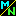Navigation Panel:(These buttons explained below)# Does the number e have any real physical meaning, or is it just a mathematical convenience?

Yes, the number e does have physical meaning. It occurs naturally in any situation where a quantity increases at a rate proportional to its value, such as a bank account producing interest, or a population increasing as its members reproduce.

Obviously, the quantity will increase more if the increase is based on the total current quantity (including previous increases), than if it is only based on the original quantity (with previous increases not counted). How much more? The number e answers this question.

To put it another way, the number e is related to the how much more money you will earn under compound interest than you would under simple interest.

Therefore, we will address the following topics:

• Simple and Compound Interest:
a discussion of the meaning of simple and compound interest, and related concepts in more general situations such as population growth.
• A Physical Meaning for the Number e:
how the concepts of simple and compound interest lead to a physical interpretation of the number e in one particular case (the case in which your money would double under simple interest).
• The General Situation:
how the number e enters in to the picture when we aren't in this one particular case.
• The Number e as a Limit:
what happens when interest is compounded only at specific times instead of continually, and how this yields a mathematical definition for the number e as a certain limit.
• The Number e in Calculus:
why the number e is an especially important and natural one in calculus.

You may select any of the items above, or follow the [Go On] links to see them discussed in order.

Navigation Panel: